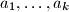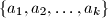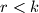### IMO Shortlist 2013 problem C4

Kvaliteta:
Avg: 0.0
Težina:
Avg: 6.5
Let$n$ be a positive integer, and$A$ be a subset of$\{1, \ldots, n\}$. An$A$-partition of$n$ into$k$ parts is a representation of$n$ as a sum$n = a_1 + \cdots a_k$, where the parts$a_1, \ldots, a_k$ belong to$A$ and are not necessarily distinct. The number of different parts in such a partition is the number of (distinct) elements in the set$\{a_1, a_2, \ldots, a_k\}$.

We say that an A-partition of$n$ into$k$ parts is optimal if there is no$A$-partition of$n$ into$r$ parts with$r < k$. Prove that any optimal$A$-partition of$n$ containts at most$\sqrt{6n}$ different parts.
Source: Germany